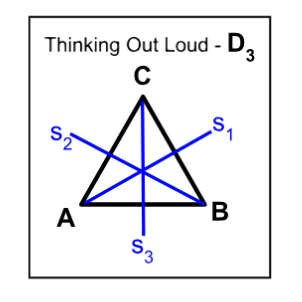# 3.3: Dihedral Groups

$$\newcommand{\vecs}{\overset { \rightharpoonup} {\mathbf{#1}} }$$ $$\newcommand{\vecd}{\overset{-\!-\!\rightharpoonup}{\vphantom{a}\smash {#1}}}$$$$\newcommand{\id}{\mathrm{id}}$$ $$\newcommand{\Span}{\mathrm{span}}$$ $$\newcommand{\kernel}{\mathrm{null}\,}$$ $$\newcommand{\range}{\mathrm{range}\,}$$ $$\newcommand{\RealPart}{\mathrm{Re}}$$ $$\newcommand{\ImaginaryPart}{\mathrm{Im}}$$ $$\newcommand{\Argument}{\mathrm{Arg}}$$ $$\newcommand{\norm}{\| #1 \|}$$ $$\newcommand{\inner}{\langle #1, #2 \rangle}$$ $$\newcommand{\Span}{\mathrm{span}}$$ $$\newcommand{\id}{\mathrm{id}}$$ $$\newcommand{\Span}{\mathrm{span}}$$ $$\newcommand{\kernel}{\mathrm{null}\,}$$ $$\newcommand{\range}{\mathrm{range}\,}$$ $$\newcommand{\RealPart}{\mathrm{Re}}$$ $$\newcommand{\ImaginaryPart}{\mathrm{Im}}$$ $$\newcommand{\Argument}{\mathrm{Arg}}$$ $$\newcommand{\norm}{\| #1 \|}$$ $$\newcommand{\inner}{\langle #1, #2 \rangle}$$ $$\newcommand{\Span}{\mathrm{span}}$$$$\newcommand{\AA}{\unicode[.8,0]{x212B}}$$

Dihedral groups are an important class of groups in abstract algebra that arise naturally in geometry and other areas of mathematics.

##### Definition: Dihedral Groups

A dihedral group is a group of symmetries of a regular polygon with n sides, where n is a positive integer. The dihedral group of order 2n, denoted by D_n, is the group of all possible rotations and reflections of the regular polygon.The group $$D_n$$ consists of $$2n$$ elements, which can be depicted as follows:

• $$n$$ rotations, denoted by $$R_0, R_{360/n}, R_{(360)(2)/n} ..., R_{(n-1)360/n},$$ where $$R_{i (360)/n}$$ represents a rotation of $$(360i/n)$$ degrees clockwise about the center of the polygon.
• $$n$$ reflections, denoted by $$F_0, F_1, F_2, ..., F_{(n-1)},$$ where $$F_i$$ represents a reflection across a line passing through the center of the polygon and one of its vertices.

The group operation in $$D_n,$$  is the composition of symmetries.

Alternatively,  the Dihedral group $$D_n$$ is defined by  $$D_n=<r,s | s^2 =e, r^n=e, srs=r^{-1}>$$ and, $$|D_n|= 2n.$$

##### Theorem $$\PageIndex{1}$$Dihedral groups  $$D_n$$ are non-abelian, for integers $$n ≥ 3.$$

Proof

Let $$a,b \in D_3$$.

We will show that $$ab \ne ba, \; \forall a,b \in D_n$$, $$n \ge 3$$.

$$D_3=\{e,(AB),(AC),(BC),(ABC),(ACB)\}$$.

Since $$D_3$$ is isomorphic to $$S_3$$, $$D_3$$ is non-abelian.

Since $$D_3 \le D_n, \forall \; n\ge 3$$, $$D_n$$ is non-abelian for $$n \ge 3$$.

The Dihedral group $$D_3$$ is same as $$S_3.$$

##### Example $$\PageIndex{1}$$

Consider the possible rotations and reflections of a  4-sided regular polygon -Square, $$D_4$$.Cayley Table for $$D_4$$ R0 R90 R180 R270 H V D D’ R0 R0 R90 R180 R270 H V D D’ R90 R90 R180 R270 R0 D’ D H V R180 R180 R270 R0 R90 V H D’ D R270 R270 R0 R90 R180 D D’ V H H H D V D’ R0 R180 R90 R270 V V D’ H D R180 R0 R270 R90 D D V D’ H R270 R90 R0 R180 D’ D’ H D V R90 R270 R180 R0

Examples abound of corporations using Dihedral groups, $$D_n,$$ as their logos. Below are examples of $$D1, D3,$$ and $$D5.$$##### Example $$\PageIndex{1}\ Consider the Dihedral group \(D_4$$.  $$D_4=<r,s|r^4=1, s^2=1, srs=r^{-1}>$$.

1. Find all the subgroups lattice of $$D_4$$, the Dihedral group of order 8.

$$D_4=\{1,r,r^2,r^3,s,rs,r^2s,r^3s\}$$. Thus the subgroup lattice would look like:2. Prove that every subgroup of $$D_4$$ of odd order is cyclic.

Proof:

We shall proceed by showing that all odd subgroups of $$D_4$$ are cyclic.

The only possible odd subgroup is $$\{e\}$$, which is cyclic.

Thus every subgroup of $$D_4$$ of odd order is cyclic.◻

##### Theorem $$\PageIndex{1}$$

Let the group $$D_n=<r,s|r^n=1, s^2=1,srs=r^{-1}>$$.

1. Then  $$r^ks=sr^{-k}$$.

Proof

Consider $$r^ks=er^ks=s^2r^ks=ssr^ks=sr^{-k}.$$

1. The order of $$r^k$$ is $$\frac{n}{\gcd(k,n)}$$.

Proof

We will first show that $$r^k$$ is a generator of $$D_n$$ if and only if $$n|k$$.

Consider $$e=r^k$$.

Then by the division algorithm, $$k=na+b$$ where $$0 \le b < n$$.

Thus $$e=r^k$$\)=r^{na+b}\)\)=r^{na}r^b\)\)=er^b\)\)=r^b\).

Since the smallest possible integer, $$m$$, such that $$r^m=e$$ is $$n$$, $$b=0$$.

Conversely, if $$n|k$$, then $$k=ns, s \in \mathbb{Z}$$.

Thus $$r^k=r^{ns}=(r^n)^s=e^s=e$$.

Thus $$r^k=e$$ iff $$n|k$$.

Let $$b=r^k, \in D_4$$ since $$r$$ is a generator of $$D_n$$.

We shall show that the smallest integer, $$m$$ s.t. $$r^k=e$$ is $$\frac{n}{k}$$.

Let $$d=\gcd(n,k)$$.

Consider $$e=b^m=r^{km}$$.

Since this is the smallest integer $$m$$ such that $$n|km$$.  Thus $$\frac{n}{d}|m(\frac{k}{d})$$.

Since $$d$$ is the greatest common divisor of $$n$$ and $$k$$, $$\frac{n}{d}$$ and $$\frac{k}{d}$$ are relatively prime.

Hence $$\frac{n}{d}|m(\frac{k}{d})$$ means that $$\frac{n}{d}|m$$.  The smallest such $$m$$ is $$\frac{n}{d}$$.

Thus $$|r^k|= \frac{n}{\gcd(n,k)}$$.◻

##### Example $$\PageIndex{1}$$

Find the centre of $$D_n$$.

Solution:

Let $$D_n=<r,s|r^n=1, s^2=1,srs=r^{-1}>$$

We shall show that $$Z(D_n)=\{x \in D_n|dx=xd, \; \forall d \in D_n\} =\big\{ \begin{array}{c} \{1\}, if n is not divisible by 4\\ \{1, r^2\} if n is not divisible by 4\\$$.

Let's consider an element $$x$$ in $$D_n$$ that commutes with every other element in the group. Therefore, any element in D_n that commutes with every other element is either the identity element e or a rotation R^k where k is a multiple of n/2 (for even n).

• If x is the identity element e, then it commutes with every element, and it is in the center.
• If x is a rotation R^k, where k is a multiple of n/2 (for even n), or x is the identity element e (for odd n), then it commutes with every element, and it is in the center.
• If x is a rotation R^k, where k is not a multiple of n/2 (for even n), then it commutes with all rotations, but not with reflections. Therefore, it cannot commute with every element, and it is not in the center.

By combining these two statements, we can conclude that the center of D_n consists of the identity element e and the rotation R^{n/2} (if n is divisible by 4), or just the identity element e (if n is not divisible by 4) for even n. For odd n, the center consists only of the identity element e.

3.3: Dihedral Groups is shared under a not declared license and was authored, remixed, and/or curated by LibreTexts.